# 一类具有时滞效应与脉冲控制的捕食–食饵系统的动力学研究Dynamic Analysis of a Predator-Prey System with Time Delay and Pulse Control

DOI: 10.12677/AAM.2018.78116, PDF, HTML, XML, 下载: 674  浏览: 851  国家自然科学基金支持

Abstract: Based on theory of ecological dynamics and ecological control modeling idea, a predator-prey dy-namic system with time-delay and pulse control is constructed. The sufficient criterion of local asymptotic stability and global attraction for the semi-trivial periodic solution of the system is es-tablished. The permanence of the system is proved. Further, the dynamic behavior of the model was simulated numerically, which verifies the validity and feasibility of theoretical analysis.

1. 引言

$\left\{\begin{array}{l}{\stackrel{˙}{N}}_{i}\left(t\right)=B\left(t\right)-{D}_{i}\left(t\right)-W\left(t\right)\\ {\stackrel{˙}{N}}_{m}\left(t\right)=\alpha W\left(t\right)-{D}_{m}\left(t\right)\end{array}$ (1)

$\left\{\begin{array}{l}\stackrel{˙}{x}\left(t\right)=\beta y\left(t\right)-rx\left(t\right)-\beta {\text{e}}^{-r\tau }y\left(t-\tau \right)\\ \stackrel{˙}{y}\left(t\right)=\beta {\text{e}}^{-r\tau }y\left(t-\tau \right)-{\eta }_{2}{y}^{2}\left(t\right)\end{array}$ (2)

$\stackrel{˙}{N}\left(t\right)=N\left(t\right)\left(r-aN\left(t\right)\right)$ (3)

$\left\{\begin{array}{l}\stackrel{˙}{x}\left(t\right)=\left[r-a\left(x\left(t\right)+y\left(t\right)\right)\right]x\left(t\right)-r{\text{e}}^{-{d}_{1}\tau }y\left(t-\tau \right)-{d}_{1}x\left(t\right)\\ \stackrel{˙}{y}\left(t\right)=r{\text{e}}^{-{d}_{1}\tau }y\left(t-\tau \right)+\left[r-{d}_{2}-a\left(x\left(t\right)+y\left(t\right)\right)\right]y\left(t\right)-by\left(t\right)z\left(t\right)\\ \stackrel{˙}{z}\left(t\right)=kby\left(t\right)z\left(t\right)-{d}_{3}z\left(t\right)\end{array}$ (4)

$\left\{\begin{array}{l}\begin{array}{l}\stackrel{˙}{x}\left(t\right)=\left[r-a\left(x\left(t\right)+y\left(t\right)\right)\right]x\left(t\right)-r{\text{e}}^{-{d}_{1}\tau }y\left(t-\tau \right)-{d}_{1}x\left(t\right)\\ \stackrel{˙}{y}\left(t\right)=r{\text{e}}^{-{d}_{1}\tau }y\left(t-\tau \right)+\left[r-{d}_{2}-a\left(x\left(t\right)+y\left(t\right)\right)\right]y\left(t\right)-by\left(t\right)z\left(t\right)\\ \stackrel{˙}{z}\left(t\right)=kby\left(t\right)z\left(t\right)-{d}_{3}z\left(t\right)\end{array}\right\}t\ne nT\\ \begin{array}{l}\Delta x\left(t\right)=0\\ \Delta y\left(t\right)=0\\ \Delta z\left(t\right)=R\end{array}\right\}t=nT\end{array}$ (5)

$\left\{\begin{array}{l}\left({\varphi }_{1},{\varphi }_{2},\psi \right)\in \left(\left[-\tau ,0\right],{R}_{3}^{+}\right),\\ x\left(t\right)={\varphi }_{1}\left(t\right),y\left(t\right)={\varphi }_{2}\left(t\right),z\left(t\right)=\psi \left(t\right),\\ {R}_{3}^{+}=\left\{x\in {R}^{3}:x\ge 0\right\}.\end{array}$ (6)

$D=\left\{\left(x\left(t\right),y\left(t\right),z\left(t\right)\right):x\left(t\right)>0,y\left(t\right)>0,z\left(t\right)>0\right\}$ (7)

2. 定理

2) V对于X满足局部的Lipschitz条件。

$\left\{\begin{array}{l}\stackrel{˙}{w}\left(t\right)\le p\left(t\right)w\left(t\right)+q\left(t\right),\text{\hspace{0.17em}}\text{\hspace{0.17em}}t\ne {\tau }_{i},\text{\hspace{0.17em}}\text{\hspace{0.17em}}t>0\\ w\left({t}^{+}\right)\le {p}_{i}w\left(t\right)+{q}_{i},\text{\hspace{0.17em}}\text{\hspace{0.17em}}t=n{\tau }_{i}>0\\ u\left({0}^{+}\right)\le {w}_{0},\end{array}$ (8)

$\begin{array}{l}w\left(t\right)\le w\left(0\right)\frac{\Pi }{0<{\tau }_{i}

$\left\{\begin{array}{l}\stackrel{˙}{h}\left(t\right)=-{d}_{3}h\left(t\right)\text{\hspace{0.17em}}\text{\hspace{0.17em}}\text{\hspace{0.17em}}\text{\hspace{0.17em}}\text{\hspace{0.17em}}t\ne nT\\ h\left({t}^{+}\right)=h\left(t\right)+R\text{\hspace{0.17em}}\text{\hspace{0.17em}}\text{\hspace{0.17em}}\text{\hspace{0.17em}}t=nT\\ h\left({0}^{+}\right)={h}_{0}\end{array}$ (9)

${h}^{*}\left(t\right)=\frac{R{e}^{-{d}_{3}\left(t-nT\right)}}{1-{\text{e}}^{-{d}_{3}T}},\text{\hspace{0.17em}}\text{\hspace{0.17em}}\text{\hspace{0.17em}}\text{\hspace{0.17em}}t\in \left(nT,\left(n+1\right)T\right]$ .

$\frac{\text{d}u}{\text{d}t}=au\left(t-\tau \right)-b\left(t\right)u\left(t\right)$ , (10)

1) 若 $a>b$ ，则 $\underset{t\to \infty }{\mathrm{lim}}u\left(t\right)=+\infty$

2) 若 $a\le b$ ，则 $\underset{t\to \infty }{\mathrm{lim}}u\left(t\right)=0$

$\frac{\text{d}u}{\text{d}t}=au\left(t-\tau \right)-bu\left(t\right)-c{u}^{2}\left(t\right)$ , (11)

1) 若 $a>b$ ，则 $\underset{t\to \infty }{\mathrm{lim}}u\left(t\right)=\frac{a-b}{c}$

2) 若 $a\le b$ ，则 $\underset{t\to \infty }{\mathrm{lim}}u\left(t\right)=0$

3. 理论分析

3.1. 系统(5)任意解的有界性

$t\ne nT$ 时，

$\begin{array}{l}{D}^{+}V\left(t\right)=k\left[r-a\left(x\left(t\right)+y\left(t\right)\right)\right]x\left(t\right)-kr{\text{e}}^{-{d}_{1}\tau }y\left(t-\tau \right)-k{d}_{1}x\left(t\right)+kr{\text{e}}^{-{d}_{1}\tau }y\left(t-\tau \right)\\ \text{\hspace{0.17em}}\text{\hspace{0.17em}}\text{\hspace{0.17em}}\text{\hspace{0.17em}}\text{\hspace{0.17em}}\text{\hspace{0.17em}}\text{\hspace{0.17em}}\text{\hspace{0.17em}}\text{\hspace{0.17em}}\text{\hspace{0.17em}}\text{\hspace{0.17em}}\text{\hspace{0.17em}}\text{\hspace{0.17em}}\text{\hspace{0.17em}}+k\left[r-{d}_{2}-a\left(x\left(t\right)+y\left(t\right)\right)\right]y\left(t\right)-kby\left(t\right)z\left(t\right)+kby\left(t\right)z\left(t\right)-{d}_{3}z\left(t\right)\\ \text{\hspace{0.17em}}\text{\hspace{0.17em}}\text{\hspace{0.17em}}\text{\hspace{0.17em}}\text{\hspace{0.17em}}\text{\hspace{0.17em}}\text{\hspace{0.17em}}\text{\hspace{0.17em}}\text{\hspace{0.17em}}\text{\hspace{0.17em}}\text{\hspace{0.17em}}=k\left[-a{x}^{2}\left(t\right)+\left(r-{d}_{1}+{d}_{3}\right)x\left(t\right)\right]-2kax\left(t\right)y\left(t\right)\\ \text{\hspace{0.17em}}\text{\hspace{0.17em}}\text{\hspace{0.17em}}\text{\hspace{0.17em}}\text{\hspace{0.17em}}\text{\hspace{0.17em}}\text{\hspace{0.17em}}\text{\hspace{0.17em}}\text{\hspace{0.17em}}\text{\hspace{0.17em}}\text{\hspace{0.17em}}\text{\hspace{0.17em}}\text{\hspace{0.17em}}\text{\hspace{0.17em}}+k\left[-a{y}^{2}\left(t\right)+\left(r-{d}_{2}+{d}_{3}\right)y\left(t\right)\right]-{d}_{3}V\left(t\right)\end{array}$

${D}^{+}V\left(t\right)+{d}_{3}V\left(t\right)\le \frac{k{\left(r-{d}_{1}+{d}_{3}\right)}^{2}}{4a}+\frac{k{\left(r-{d}_{2}+{d}_{3}\right)}^{2}}{4a}=\frac{k\left[{\left(r-{d}_{1}+{d}_{3}\right)}^{2}+{\left(r-{d}_{2}+{d}_{3}\right)}^{2}\right]}{4a}={M}_{0}$

$\left\{\begin{array}{l}{D}^{+}V\left(t\right)\le -{d}_{3}V\left(t\right)+{M}_{0}\text{\hspace{0.17em}}\text{\hspace{0.17em}}\text{\hspace{0.17em}}\text{\hspace{0.17em}}t\ne nT\\ V\left({t}^{+}\right)=V\left(t\right)+R\text{\hspace{0.17em}}\text{\hspace{0.17em}}\text{\hspace{0.17em}}\text{\hspace{0.17em}}\text{\hspace{0.17em}}t=nT\end{array}$ (12)

$\begin{array}{l}V\left(t\right)\le \left[V\left({0}^{+}\right)-\frac{{M}_{0}}{{d}_{3}}\right]{\text{e}}^{-{d}_{3}t}+\frac{R{e}^{-{d}_{3}\left(t-nT\right)}\left(1-{\text{e}}^{-{d}_{3}nT}\right)}{1-{\text{e}}^{-{d}_{3}T}}+\frac{{M}_{0}}{{d}_{3}}\text{\hspace{0.17em}}\text{\hspace{0.17em}}\text{\hspace{0.17em}}\text{\hspace{0.17em}}t\in \left(nT,\left(n+1\right)T\right]\\ \text{\hspace{0.17em}}\text{\hspace{0.17em}}\text{\hspace{0.17em}}\text{\hspace{0.17em}}\text{\hspace{0.17em}}\text{\hspace{0.17em}}\text{\hspace{0.17em}}\le \frac{R{e}^{{d}_{3}T}}{1-{\text{e}}^{-{d}_{3}T}}+\frac{{M}_{0}}{{d}_{3}}\triangleq M\text{\hspace{0.17em}}\text{\hspace{0.17em}}\text{\hspace{0.17em}}\text{\hspace{0.17em}}\text{\hspace{0.17em}}\left(t\to \infty \right)\end{array}$ (13)

3.2. 系统(5)半平凡周期解的局部渐近稳定性

$\left\{\begin{array}{l}\begin{array}{l}\stackrel{˙}{H}\left(t\right)=\left(r-{d}_{1}\right)H\left(t\right)-r{\text{e}}^{-{d}_{1}\tau }P\left(t\right)\\ \stackrel{˙}{P}\left(t\right)=\left[r-{d}_{2}-b{z}^{*}\left(t\right)+r{\text{e}}^{-{d}_{1}\tau }\right]P\left(t\right)\\ \stackrel{˙}{W}\left(t\right)=kb{z}^{*}\left(t\right)P\left(t\right)-{d}_{3}W\left(t\right)\end{array}\right\}t\ne nT\\ \begin{array}{l}H\left({t}^{+}\right)=H\left(t\right)\\ P\left({t}^{+}\right)=P\left(t\right)\\ W\left({t}^{+}\right)=W\left(t\right)+R\end{array}\right\}t=nT\end{array}$ (14)

$\frac{\text{d}\varphi \left(t\right)}{\text{d}t}=A\left(t\right)\varphi \left(t\right)$ (15)

$t=nT$ 时， $\left[\begin{array}{c}H\left(n{T}^{+}\right)\\ P\left(n{T}^{+}\right)\\ W\left(n{T}^{+}\right)\end{array}\right]=\left[\begin{array}{ccc}1& 0& 0\\ 0& 1& 0\\ 0& 0& 1\end{array}\right]\left[\begin{array}{c}H\left(nT\right)\\ P\left(nT\right)\\ W\left(nT\right)\end{array}\right]$

$\Phi \left(T\right)=\varphi \left(0\right){\text{e}}^{\underset{0}{\overset{T}{\int }}A\left(s\right)\text{d}s}\triangleq \varphi \left(0\right)\mathrm{exp}\left(\stackrel{˜}{A}\right),\text{\hspace{0.17em}}\stackrel{˜}{A}=\underset{0}{\overset{T}{\int }}A\left(s\right)\text{d}s$ (16)

${\lambda }_{1}={\text{e}}^{\underset{0}{\overset{T}{\int }}\left(r-{d}_{1}\right)\text{d}s},\text{\hspace{0.17em}}{\lambda }_{2}={\text{e}}^{\underset{0}{\overset{T}{\int }}\left[r-{d}_{2}-b{z}^{*}\left(s\right)+r{\text{e}}^{-{d}_{1}\tau }\right]\text{d}s},\text{\hspace{0.17em}}{\lambda }_{3}={\text{e}}^{\underset{0}{\overset{T}{\int }}\left(-{d}_{3}\right)\text{d}s}={\text{e}}^{-{d}_{3}T}<1$ (17)

$z\left(t\right)=\left[{z}_{0}-\frac{R}{1-{\text{e}}^{-{d}_{3}T}}\right]{\text{e}}^{-{d}_{3}t}+{z}^{*}\left(t\right),\text{\hspace{0.17em}}t\in \left(nT,\left(n+1\right)T\right]$ . (18)

3.3. 系统(5)半平凡周期解的全局吸引性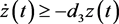(19)(20)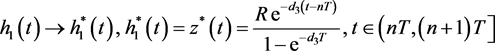. (21)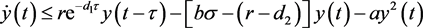(22)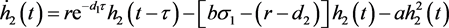(23)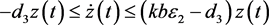(24)(25)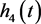是系统(26)的解。(26)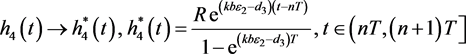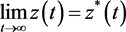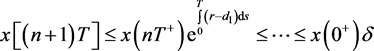(27)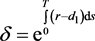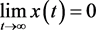3.4. 系统(5)的持久生存性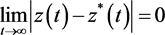，知对任意的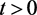，存在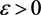，使得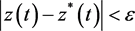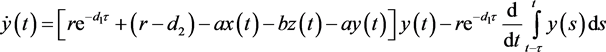(28)(29)

(H1)：假设对上面的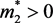，对任意的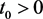，当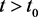时，有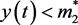不恒成立，则可以假设存在，使得当时有成立。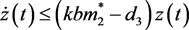(30)(31)(32)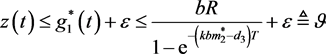(33)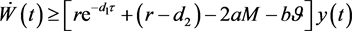(34)(35)

(H2)：根据第一个假设，只需要考虑两种情况，

1)对所有的充分大的t，都有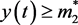成立；

2)当t充分大时，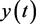关于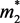左右波动。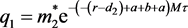(36)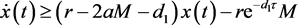(37)

4. 数值模拟

5. 结论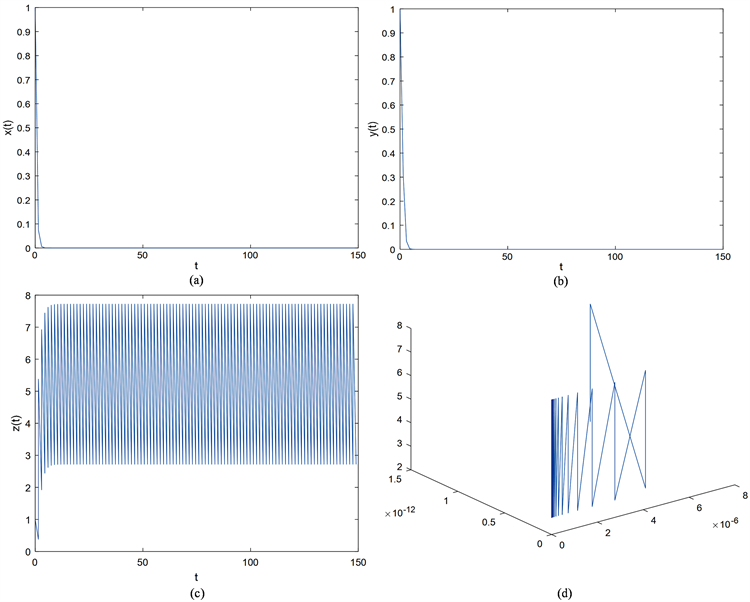Figure 1. Time diagram and phase diagram of system (5) when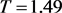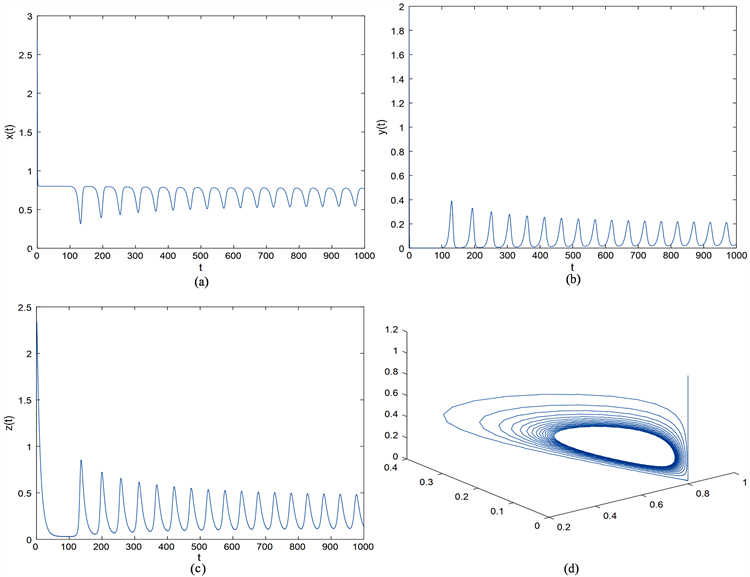Figure 2. Time diagram and phase diagram of system (5) when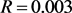宋燕, 杜月, 李紫薇. 具有阶段结构及脉冲控制的害虫管理模型[J]. 渤海大学学报(自然科学版), 2017, 38(2): 104-110.  Cai, S., Jiao, J. and Li, L. (2015) Dynamics of a Pest Management Predator-Prey Model with Stage Structure and Im-pulsive Stocking. Journal of Applied Mathematics & Computing, 52, 125-138. https://doi.org/10.1007/s12190-015-0933-3  Grasman, J., van Herwaarden, O.A., Hemerik, L. and van Lenteren, J.C. (2001) A Two-Component Model of Host-Parasitoid Interactions: Determination of the Size of Inundative Releases of Parasitoids in Biological Pest Control. Mathematical Biosciences, 169, 207-216. https://doi.org/10.1016/S0025-5564(00)00051-1  Liu, X. and Chen, L. (2003) Complex Dynamics of Holling Type ii Lotka-Volterra Predator-Prey System with Impulsive Perturbations on the Predator. Chaos Solitons & Fractals, 16, 311-320. https://doi.org/10.1016/S0960-0779(02)00408-3  Panetta, J.C. (1996) A Mathematical Model of Periodically Pulsed Che-motherapy: Tumor Recurrence and Metastasis in a Competitive Environment. Bulletin of Mathematical Biology, 58, 425-447. https://doi.org/10.1007/BF02460591  Barclay, H.J. (1982) Models for Pest Control Using Predator Release, Habitat Man-agement and Pesticide Release in Combination. Journal of Applied Ecology, 19, 337-348. https://doi.org/10.2307/2403471  Lotka, A.J. (1927) Scientific Books: Elements of Physical Biology. Science, 66, 281-282. https://doi.org/10.1126/science.66.1708.281-a  Hethcote, H.W., Wang, W., Han, L. and Ma, Z. (2004) A Predator-Prey Model with Infected Prey. Theoretical Population Biology, 66, 259-268. https://doi.org/10.1016/j.tpb.2004.06.010  Liu, S. and Beretta, E. (2006) A Stage-Structured Predator-Prey Model of Beddington-Deangelis Type. Siam Journal on Applied Mathematics, 66, 1101-1129. https://doi.org/10.1137/050630003  Gourley, S.A. and Lou, Y. (2014) A Mathematical Model for the Spatial Spread and Biocontrol of the Asian Longhorned Beetle. Siam Journal on Applied Mathematics, 74, 2-11. https://doi.org/10.1137/130939304  Yu, H., Zhao, M., Wang, Q. and Agarwal, R.P. (2014) A Focus on Long-Run Sustai-nability of an Impulsive Switched Eutrophication Controlling System Based upon the Zeya Reservoir. Journal of the Franklin Institute, 351, 487-499. https://doi.org/10.1016/j.jfranklin.2013.08.025  Zhang, Y., Chen, S., Gao, S. and Wei, X. (2017) Stochastic Periodic Solution for a Perturbed Non-Autonomous Predator-Prey Model with Generalized Nonlinear Harvesting and Impulses. Physica A: Statistical Mechanics & Its Applications, 486, 347-366. https://doi.org/10.1016/j.physa.2017.05.058  Yu, H., Zhong, S. and Agarwal, R.P. (2011) Mathematics Analysis and Chaos in an Ecological Model with an Impulsive Control Strategy. Communications in Nonlinear Science & Numerical Simulation, 16, 776-786. https://doi.org/10.1016/j.cnsns.2010.04.017  Xie, Y., Wang, L., Deng, Q. and Wu, Z. (2017) The Dynamics of an Impulsive Predator-Prey Model with Communicable Disease in the Prey Species Only. Applied Mathematics & Computation, 292, 320-335. https://doi.org/10.1016/j.amc.2016.07.042  Wang, Z., Shao, Y., Fang, X. and Ma, X. (2016) The Dynamic Behaviors of One-Predator Two-Prey System with Mutual Interference and Impulsive Control. Mathematics & Computers in Simulation, 132, 68-85. https://doi.org/10.1016/j.matcom.2016.06.007  Ding, X. and Jiang, J. (2009) Periodicity in a Generalized Semi-Ratio-Dependent Predator-Prey System with Time Delays and Impulses. Journal of Mathematical Analysis & Applications, 360, 223-234. https://doi.org/10.1016/j.jmaa.2009.06.048  Pei, Y., Liu, S., Li, C. and Chen, L. (2009) The Dynamics of an Impulsive Delay Si Model with Variable Coefficients. Applied Mathematical Modelling, 33, 2766-2776. https://doi.org/10.1016/j.apm.2008.08.011  Tan, R., Liu, Z. and Cheke, R.A. (2012) Periodicity and Stability in a Sin-gle-Species Model Governed by Impulsive Differential Equation. Applied Mathematical Modelling, 36, 1085-1094. https://doi.org/10.1016/j.apm.2011.07.056  Dai, C., Zhao, M. and Chen, L. (2012) Complex Dynamic Behavior of Three-Species Ecological Model with Impulse Perturbations and Seasonal Disturbances. Mathematics & Computers in Simulation, 84, 83-97. https://doi.org/10.1016/j.matcom.2012.09.004  卢肠. 几类捕食-食饵模型的定性分析[J]. 哈尔滨: 哈尔滨工业大学, 2017.  Khajanchi, S. and Banerjee, S. (2017) Role of Constant Prey Refuge on Stage Structure Predator-Prey Model with Ratio Dependent Functional Response. Applied Mathematics & Computation, 314, 193-198. https://doi.org/10.1016/j.amc.2017.07.017  Jiao, J. and Chen, L. (2008) Global Attractivity of a Stage-Structure Variable Coefficients Predator-Prey System with Time Delay and Impulsive Perturbations on Predators. International Journal of Biomathe-matics, 1, 197-208. https://doi.org/10.1142/S1793524508000163  Zhang, H. and Chen, L. (2008) A Model for Two Species with Stage Structure and Feedback Control. International Journal of Biomathematics, 1, 267-286. https://doi.org/10.1142/S1793524508000230  Shao, Y. and Ma, X. (2017) Analysis of a Predator-Prey System with Bed-dington-Type Functional Response and Stage-Structure of Prey. International Journal of Dynamical Systems & Differential Equations, 7, 51. https://doi.org/10.1504/IJDSDE.2017.083727  Sailer, R.I. (1975) Book Review: Biological Control by Natural Ene-mies.  Chen, F., Xie, X. and Li, Z. (2012) Partial Survival and Extinction of a Delayed Predator-Prey Model with Stage Structure. Applied Mathematics & Computation, 219, 4157-4162. https://doi.org/10.1016/j.amc.2012.10.055  Aiello, W.G. and Freedman, H.I. (1990) A Time-Delay Model of Single-Species Growth with Stage Structure. Mathematical Biosciences, 101, 139. https://doi.org/10.1016/0025-5564(90)90019-U  Pei, Y., Li, C. and Fan, S. (2013) A Mathematical Model of a Three Species Prey-Predator System with Impulsive Control and Holling Functional Response. Applied Mathematics and Computation, 219, 10945-10955. https://doi.org/10.1016/j.amc.2013.05.012  Pang, G., Wang, F. and Chen, L. (2009) Extinction and Permanence in Delayed Stage-Structure Predator-Prey System with Impulsive Effects. Chaos Solitons & Fractals, 39, 2216-2224. https://doi.org/10.1016/j.chaos.2007.06.071  Bainov, D.D. and Simeonov, P.S. (1995) Impulsive Differential Equations: Asymptotic Properties of the Solutions. World Scientific, Singapore. https://doi.org/10.1142/2413